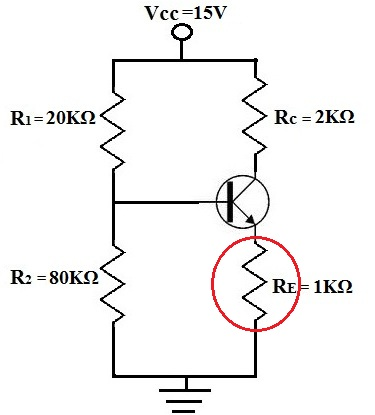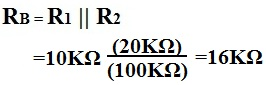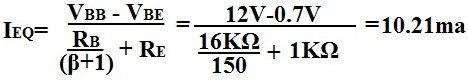﻿ How to Counter the Differences in β (Beta) from Transistor to Transistor

# How To Counter the Differences in β from Transistor to Transistor

β, the gain or amplification factor of transistors, can vary by large amounts even in the same exact type of transistors from the same batch. There is no way to replicate the same exact βs across transistor to transistor. Therefore, when we build a transistor circuit, we must make sure to build one that produces roughly the same gain despite the fluctations in β we may have in transistors.

The way to fight against this is the use of the resistor RE in a transistor circuit:Let's work out the example above and show how RE fights against the different βs of transistors and still allows for roughly the same current gain:

For the circuit above,Now let's say for one transistor β=100. Then,Now let's say that another transistor has a much wider transistor β of 150. Then,So even though there is a difference of 50 of β in the transistors, there is less than a half of milliampere difference in the output current, Ie. If wanted, an even larger value of RE can be used so that there is even more stability against the β of transistors. However, realize that the larger RE is, the more gain that is lost for amplification in the circuit because the value of Ie. So there must be balance in the design of the value of RE. A good design rule is to make RE 10 times the value of the value of RB/(β+1). So RE= 10(RB/(β+1). That's enough (usually the standard) to fight against the variation of β from transistor to transistor.